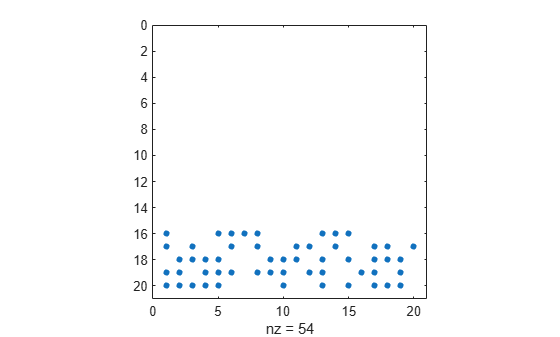spalloc

Allocate space for sparse matrix

Description

example

S = spalloc(m,n,nz) creates an all-zero sparse matrix S of size m-by-n with room to hold nz nonzero elements, where nz >= 1.

Examples

collapse all

Use spalloc to initialize a 10-by-10 all-zero sparse matrix with room for up to 20 nonzero elements.

S = spalloc(10,10,20);

Define several elements in the matrix.

S(1:3,1:3) = magic(3)
S =
(1,1)        8
(2,1)        3
(3,1)        4
(1,2)        1
(2,2)        5
(3,2)        9
(1,3)        6
(2,3)        7
(3,3)        2

Show the number of nonzero elements in the matrix.

n1 = nnz(S)
n1 = 9

Show the amount of storage allocated for nonzero matrix elements.

n2 = nzmax(S)
n2 = 20

Use spalloc to initialize a 20-by-20 all-zero sparse matrix with space for 100 nonzero elements.

n = 20;
S = spalloc(n,n,5*n);

Then use a for loop to fill in the columns of S one at a time with an average of at most five nonzero elements per column.

for j = 1:n
S(:,j) = [zeros(n-5,1); round(rand(5,1))];
end

Plot the sparsity pattern of matrix S. The dots represent the nonzero elements.

spy(S)Show the number of nonzero elements in the matrix.

n1 = nnz(S)
n1 = 54

Show the amount of storage allocated for nonzero matrix elements.

n2 = nzmax(S)
n2 = 100

Input Arguments

collapse all

Number of matrix rows, specified as a nonnegative integer.

Data Types: single | double | int8 | int16 | int32 | int64 | uint8 | uint16 | uint32 | uint64 | logical

Number of matrix columns, specified as a nonnegative integer.

Data Types: single | double | int8 | int16 | int32 | int64 | uint8 | uint16 | uint32 | uint64 | logical

Storage allocation for nonzero elements, specified as a nonnegative integer. If you specify a value of 0 for nz, then spalloc instead sets the value of nz to 1.

Data Types: single | double | int8 | int16 | int32 | int64 | uint8 | uint16 | uint32 | uint64 | logical

Limitations

• Both matrix dimensions, m and n, must be smaller than 2^31-1 on 32-bit platforms, or 2^48-1 on 64-bit platforms.

Tips

• When you assign several times into a matrix you created with spalloc, the preallocated memory can prevent repeated reallocations. However, assigning into a sparse matrix is still a relatively expensive operation, which should usually be avoided if it can easily be replaced by one of the following:

• a one-time call to the sparse function

• a one-time call to the spdiags function

• a one-time concatenation of a set of matrices, which can be sparse, dense, or both